# Texas Go Math Grade 2 Lesson 16.5 Answer Key Choose a Tool

Refer to our Texas Go Math Grade 2 Answer Key Pdf to score good marks in the exams. Test yourself by practicing the problems from Texas Go Math Grade 2 Lesson 16.5 Answer Key Choose a Tool.

## Texas Go Math Grade 2 Lesson 16.5 Answer Key Choose a Tool

Explore

Draw or write to describe how you measured the distances with the yarn.
Distance 1
Distance 2

FOR THE TEACHER • Have each small group use a 1-yard piece of yarn to measure a distance marked on the floor with masking tape. Have groups repeat the activity to measure another distance that is different from the first one.

Math Talk
Mathematical Processes

Which distance was longer? Explain how you know.

Model and Draw

These are tools for measuring lengths and distances.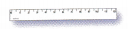inch ruler
An inch ruler can be used to measure shorter lengths.yardstick
A yardstick shows 3 feet. It can be used to measure greater lengths and distances.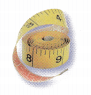measuring tape
A measuring tape can be used to measure lengths and distances that are not flat or straight.

Share and Show

Choose the best tool for measuring the real object. Then measure and record the length or distance.

inch ruler
yardstick
measuring tape

Question 1.
the length of a bookTool: __________________
Length: _________________
Tool: inch ruler
Length: 6 inches

Explanation:
I measured the length of the book using an inch ruler as an inch ruler can be used to measure shorter lengths.

Question 2.
the distance around a cupTool: __________________
Distance: _________________
Tool: Measuring tape
Length: 8 inches

Explanation:
I measured the distance around a cup by using a measuring tape as it can be used to measure lengths and distances that are not flat or straight.

Question 3.
the length of a chalkboardTool: __________________
Length: _________________
Tool: Yard stick
Length: 2 yards

Explanation:
I measured the length of a chalkboard using a yardstick as it can be used to measure greater lengths and distances.

Problem Solving

Choose the best tool for measuring the real object. Then measure and record the length or distance.

inch ruler
yardstick
measuring tape

Question 4.
the length of a markerTool: __________________
Length: _________________
Tool: inch ruler
Length: 8 inches

Explanation:
I measured the length of the book using an inch ruler as an inch ruler can be used to measure shorter lengths.

Question 5.
the distance around a globe.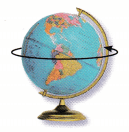Tool: __________________
Distance: _________________
Tool: Measuring tape
Length: 15 cm

Explanation:
I measured the distance around a cup by using a measuring tape as it can be used to measure lengths and distances that are not flat or straight.

Question 6.
H.O.T. Rachel wants to measure the length of a sidewalk. Should she use an inch ruler or a yardstick? Explain.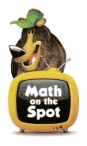Rachel should use _________ because ___________
Rachel should use yardstick because it can be used to measure greater lengths and distances.

Question 7.
H.O.T. Multi-Step What is an object that you would measure with a measuring tape? Explain why you would use this tool.
I will measure a ball using a measuring tape. I will use the tape to measure because a measuring tape can be used to measure lengths and distances that are not flat or straight.

Question 8.
Reasoning Tammy is measuring a clip that is 2 inches long. Which is the best tool for Tammy to use?
(A) an inch ruler
(B) a yardstick
(C) a piece of string
(A) an inch ruler

Explanation:
Tammy can measure the length of the clip by using an inch ruler as an inch ruler can be used to measure shorter lengths.

Question 9.
Connect Alex wants to measure the distance around a beach ball. Which is the best tool for Alex to use?
(A) an inch ruler
(B) a yardstick
(C) a measuring tape
(C) a measuring tape

Explanation:
Alex can measure the distance around a beach ball by using a measuring tape as it can be used to measure lengths and distances that are not flat or straight.

Question 10.
Brian wants to measure the fence around the park. Which is the best tool for Brian to use?
(A) a yardstick
(B) an inch ruler
(C) a color tile
(A) a yardstick

Explanation:
Brian should measure the length of a fence using a yardstick as it can be used to measure greater lengths and distances.

Question 11.
Texas Test Prep Jim wants to measure some books to find a book that is q inches long. Which ¡s the best tool for Jim to use?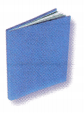(A) cup
(B) yarn
(C) inch ruler
(C) inch ruler

Explanation:
Jim can measure the length of the book by using an inch ruler as an inch ruler can be used to measure shorter lengths.

TAKE HOME ACTIVITY • Hove your child name some objects that he or she would measure using o yardstick.

### Texas Go Math Grade 2 Lesson 16.5 Homework and Practice Answer Key

Choose the best tool for measuring the real object. Then measure and record the length or distance.

Question 1.
the length of a toy car5 yards

Explanation:
I measured the length of a car using a yardstick as it can be used to measure greater lengths and distances. The real car is about 5 yards long.

Question 2.
the length of a table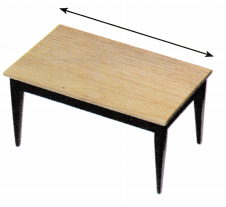40 inches

Explanation:
I measured the length of the table using an inch ruler as an inch ruler can be used to measure shorter lengths. The real table is 40 inches long.

Problem Solving

Question 3.
Multi-Step Fran wants to measure the distance around a beach ball. Should she use an inch ruler or measuring tape? Explain.
Fran should use ____ because ____Fran should use a measuring tape because it can be used to measure lengths and distances that are not flat or straight.

Lesson Check

Question 4.
Dan wants to measure some rope to find a piece that is 8 inches long. Which is the best tool for Dan to use?
(A) cup
(B) inch ruler
(C) yarn
(B) inch ruler

Explanation:
Dan can measure the length of the rope by using an inch ruler as an inch ruler can be used to measure shorter lengths.

Question 5.
Alicia wants to measure the height of her tomato plants. Which is the best tool for Alicia to use?
(A) yarn
(B) inch ruler
(C) color tile
(A) yarn

Explanation:
Alicia can use yarn to measure the height of the tomato plant as it will be easy to measure the things that are not flat or straight.

Question 6.
Tim wants to measure around a soccer ball. Which is the best tool for Tim to use?
(A) yardstick
(B) inch ruler
(C) measuring tape
(C) measuring tape

Explanation:
Tim can measure the distance around the soccer ball by using a measuring tape as it can measure lengths and distances that are not flat or straight.

Question 7.
Ruby wants to buy fabric to make curtains. She wants the width of the fabric to be 24 inches. Which is the best tool for Ruby to use to measure the width?
(A) yardstick
(B) color tile
(C) cup